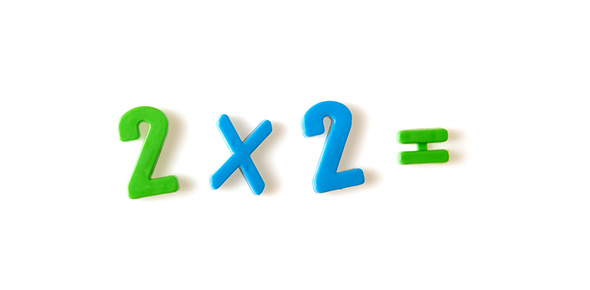# Number (Multiplication & Division) - Year 4

50 Questions | Total Attempts: 315SettingsCreate your own Quiz• 1.
Type the answer to the timestable below:  *  6 x 2 =
• 2.
Type the answer to the timestable below:  *  2 x 5 =
• 3.
Type the answer to the timestable below:  *  7 x 3 =
• 4.
Type the answer to the timestable below:  *  6 x 4 =
• 5.
Type the answer to the timestable below:  *  4 x 2 =
• 6.
Type the answer to the timestable below:  *  9 x 5 =
• 7.
Type the answer to the timestable below:  *  11 x 7 =
• 8.
Type the answer to the timestable below:  *  4 x 4 =
• 9.
Type the answer to the timestable below:  *  9 x 4 =
• 10.
Type the answer to the timestable below:  *  11 x 0 =
• 11.
Type the answer to the timestable below:  *  4 x 12 =
• 12.
Type the answer to the timestable below:  *  12 x 5 =
• 13.
Type the answer to the timestable below:  *  8 x 6 =
• 14.
Type the answer to the timestable below:  *  9 x 7 =
• 15.
Type the answer to the timestable below:  *  8 x 12 =
• 16.
Type the answer to the timestable below:  *  6 x 8 =
• 17.
Type the answer to the timestable below:  *  9 x 9 =
• 18.
Type the answer to the timestable below:  *  11 x 12 =
• 19.
Type the answer to the timestable below:  *  16 x 8 =
• 20.
Type the answer to the timestable below:  *  18 x 5 =
• 21.
Type the answer to the multi-step multiplication below:  *  3 x 5 x 4 =
• 22.
Type the answer to the multi-step multiplication below:  *  3 x 3 x 3 =
• 23.
Type the answer to the multi-step multiplication below:  *  8 x 2 x 5 =
• 24.
Type the answer to the multi-step multiplication below:  *  7 x 7 x 0 =
• 25.
Type the answer to the multi-step multiplication below:  *  12 x 9 x 2 =
Related TopicsBack to top
×

Wait!
Here's an interesting quiz for you.